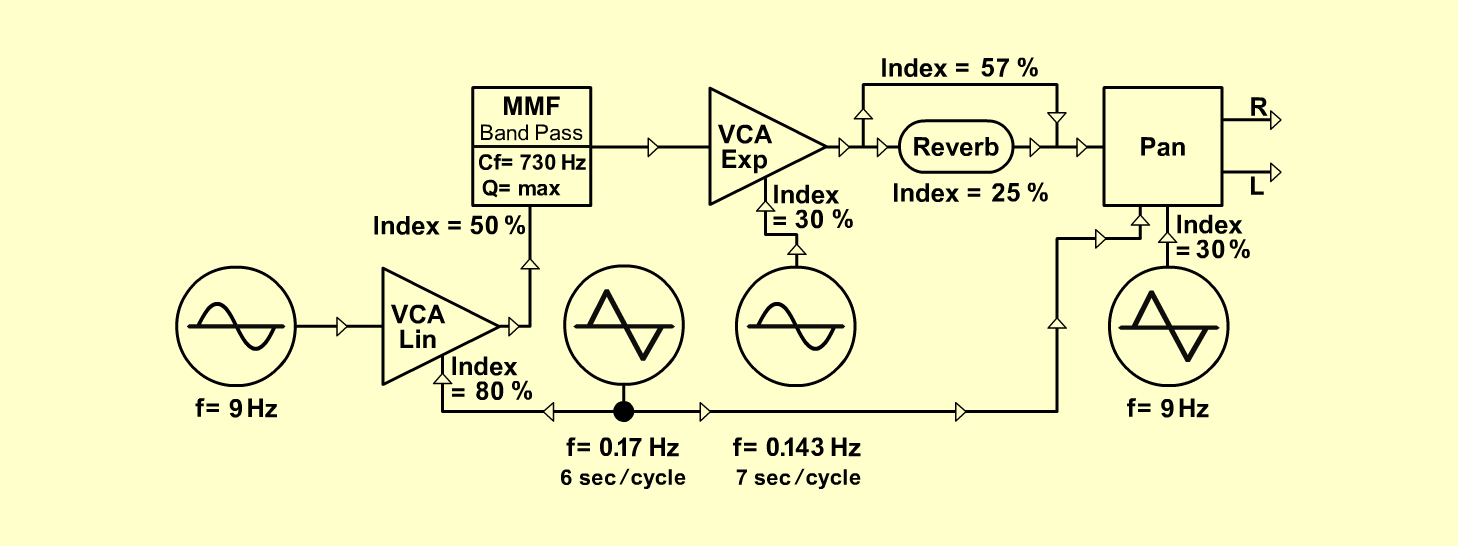Baba-YagaFXD o e p f e r A - 100 Connections: Settings: A-145 (Sine) <=>  A-130 (Audio In 1)   A-145 (Triangle) <=>  A-134 (CV 2)   A-146 (Triangle) <=>  A-180   A-180 <=>  A-130 (CV 2)   A-180 <=>  A-134 (CV 1)   A-147 (Sine) <=>  A-131 (CV 2)   A-130 (Out) <=>  A-121 (FCV 2)   A-121 (Band) <=>  A-131 (Audio In 1)   A-131 (Out) <=>  A-199 (Audio In)   A-199 (Mix Out)  <=>  A-134 (Audio In 1)   A-134 (L/R Output) <=> Amplifier A-121 (Audio Level = 10, Freq = 6, FCV 2 = 5,   Res = 10)   A-130 (Gain = 10, CV 2 = 8, Audio In 1 = 10,   Audio Out = 10)   A-131 (Gain = 10, CV 2 = 3, Audio In 1 = 10,   Audio Out = 10)   A-134 (Pan = 5, CV 2 = 3, Lev 1 = 10, Lev 2 = 10)   A-145 (Frq = 7.5, Range = M)   A-146 (Freq = 2, Wave Form = 5, Range = M)   A-147 (Freq = 3)   A-199 (Lev= 5, Feedb = 0, Emph = 0, Mix = 7.7) Josef MuellerSound sample Bab-Yaga